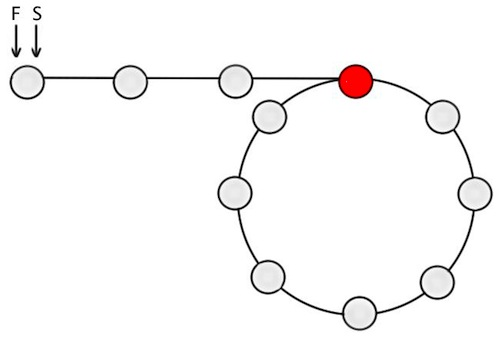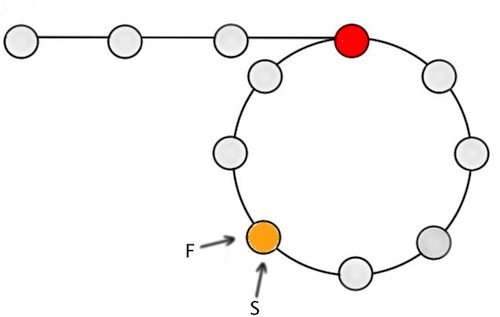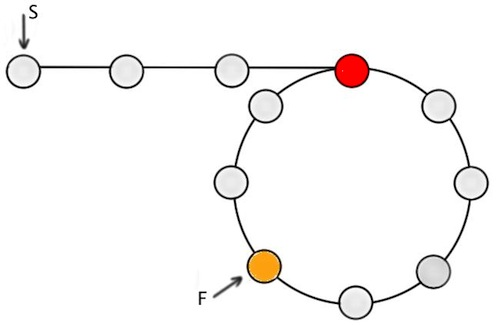# Finding the Start of a Loop in a Circular Linked List

A lot of people are familiar with the problem of detecting a loop in a linked list. The problem goes as follows: “Given a linked list, what is the algorithm to determine if it has any cycles (loops)?”

The algorithm is pretty straightforward:

1. We start at the beginning of the linked list with two pointers.
2. The first pointer is incremented through each node of the list. The second pointer moves twice as fast, and skips every other node.
3. If the linked list contains a loop, these two pointers will eventually meet at the same node, thus indicating that the linked list contains a loop.

The algorithm is straightforward and it is relatively easy to create a mental model and get an intuitive sense of why it works.

Now, a slight twist to the same question asks: “Given a circular linked list, what is the algorithm to find the first node of the loop.”

For instance, in the circular list `A->B->C->D->E->C`, the first node of the loop is node C. The first part of the algorithm is identical to the algorithm for finding if there is a loop (above). Once a loop has been found, the following additional steps will give us the starting node of the loop:

1. Once a loop as been detected (step-3 above), move one of the pointers to the beginning (head) of the linked list. The second pointer remains where it was at the end of step-3.
2. Increment both pointers one node at a time. The node at which the two pointers meet will be the starting node of the loop!

This algorithm isn’t too difficult compared to the algorithm for detecting a loop. However, the mental model seems a bit trickier. Why and how does it always find the start of the loop?

## How does the Algorithm work? An intuitive explanation

Here’s some explanation which would hopefully help you intuitively understand why the algorithm works, without going into a lot of mathematical detail.

### First, meeting point of two pointers in a loop

Consider two pointers: a slow pointer `S` that increments by one node at each step, and a fast pointer `F` that increments by two nodes at each step (i.e. it is twice as fast as `S`). Both pointers start at the same time from the beginning of an n-node loop. In the time `S` covers n nodes. `F` will have covered `2n` nodes and they will both meet at the start of the loop.

Now, let us say that the slow pointer `S` starts at the beginning of the loop, and the fast pointer `F` starts at node k (where k < n) of the loop. As these two pointers move along the loop, they will meet at node (n-x).

What we really need to do is figure out x, as it will give us the node at which the two pointers meet inside the loop.

1. When `S` takes `n/2` steps, it will be at node `n/2`. During the same time, `F` will have taken `2(n/2) = n` steps, and it will be at node `(k+n)`. Since the we are inside a loop, `F` will be effectively back at node `k`.
2. In order for the two pointers to meet at node `(n-x)`, `S` needs to take a further `(n-x-n/2)=(n/2-x)` steps and it will end up at node `n-x`. During the same time, `F` will have taken 2*(n/2-x)=(n-2x) steps and will be at node `k+(n-2x)`. Given our assumption that both S and `F` meet at the same node:

``````    n-x = k+n-2x
=>    x = k``````

This means that if S starts from the start of the loop, and F starts k nodes into the loop, both of them will meet at node (n-k), i.e k nodes from the end of the loop. This is a key insight.

## Circular Linked List

Now, coming back to the linked list that contains a loop. Suppose the start of the loop is `m` (e.g. `m`=3) nodes from the start of the linked list. Both `S` and `F` start at the beginning of the linked list [Figure-1].Figure-1: Circular linked list with S and F pointers at the start

By the time `S` gets to node `m` (i.e. start of loop), `F` will be at node `2m` [Figure-2]. This means that `S` will be at the start of the loop and `F` will be `m` nodes into the loop.Figure-2: Circular linked list, with S at the start of loop and F m nodes into the loop

Based on the discussion above, we already know that if `S` begins from the start of the loop and `F` starts from node `m`, they will meet `m` nodes from the end of the loop (i.e. the orange-node in [Figure-3]).Figure-3: Both F and S meet m nodes from the end of the loop

At this point, keep the pointer `F` at the orange-node where the two pointers met (i.e. `m`-nodes from the start of the loop), and move the pointer `S` to the beginning of the linked list [Figure-4]. Now, if we increment both `S` and `F` one node at a time, it is obvious that they will meet at ‘Node-m’ (red-node) of the list, which is the start of the loop.

{% img center /images/circular-loop-figure-4.jpg Figure-4 %}Figure-4: S at the start of linked list, F at the point they met. Both increment one at a time from here-on

For the curious, here’s the Java code snippets for detecting a loop in a linked list and finding the starting node:

``````/**
* Checks if the given linked list is a circular linked list (i.e. it
* contains a loop). This means a list in which a node's next pointer points
* to an earlier node, so as to make a loop in the linked list. For
* instance:
*     A -> B -> C -> D -> E -> C
*
*            the linked list to be tested
* @return true if there is a loop, false if there isn't
*/
return false;
}

while (true) {
slow = slow.getNext();

if (fast.getNext() != null) {
fast = fast.getNext().getNext();
} else {
return false;
}

if (slow == null || fast == null) {
return false;
}

if (slow == fast) {
return true;
}
}
}``````
``````/**
* Returns the node at the start of a loop in the given circular linked
* list. A circular list is one in which a node's next pointer points
* to an earlier node, so as to make a loop in the linked list. For
* instance:
*
* input: A -> B -> C -> D -> E -> C [the same C as earlier]
* output: C
*
* (CCI_0205)
*
*            list to be tested
* @return the node at the start of the loop if there is a loop, null
* otherwise
*/
return null;
}

IntegerNode loopStartNode = null;

while (slow != null && fast != null) {
slow = slow.getNext();
if (fast.getNext() == null) {
loopStartNode = null;
break;
}
fast = fast.getNext().getNext();

// If slow and fast point to the same node, it means that the
// linkedList contains a loop.
if (slow == fast) {

while (slow != fast) {
// Keep incrementing the two pointers until they both
// meet again. When this happens, both the pointers will
// point to the beginning of the loop
slow = slow.getNext(); // Can't be null, as we have a loop
fast = fast.getNext(); // Can't be null, as we have a loop
}

loopStartNode = slow;
break;
}
}

return loopStartNode;
}``````